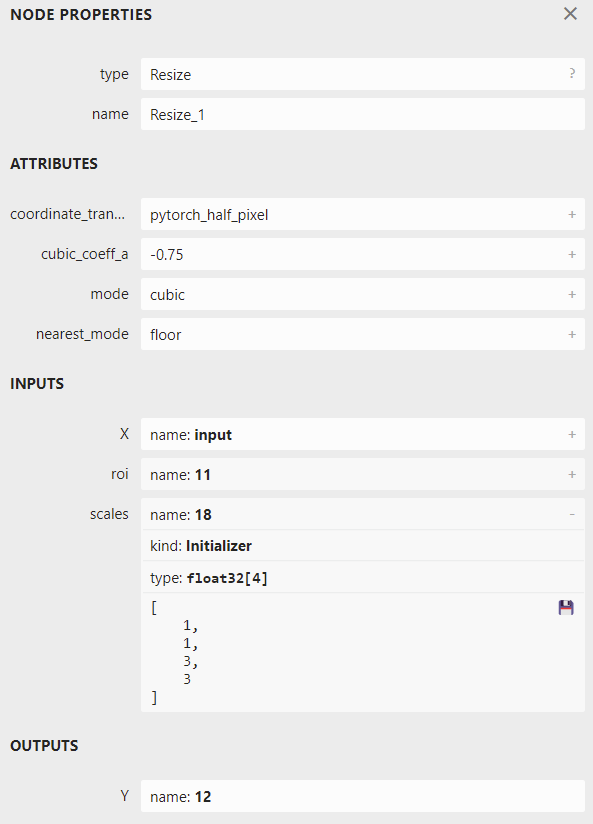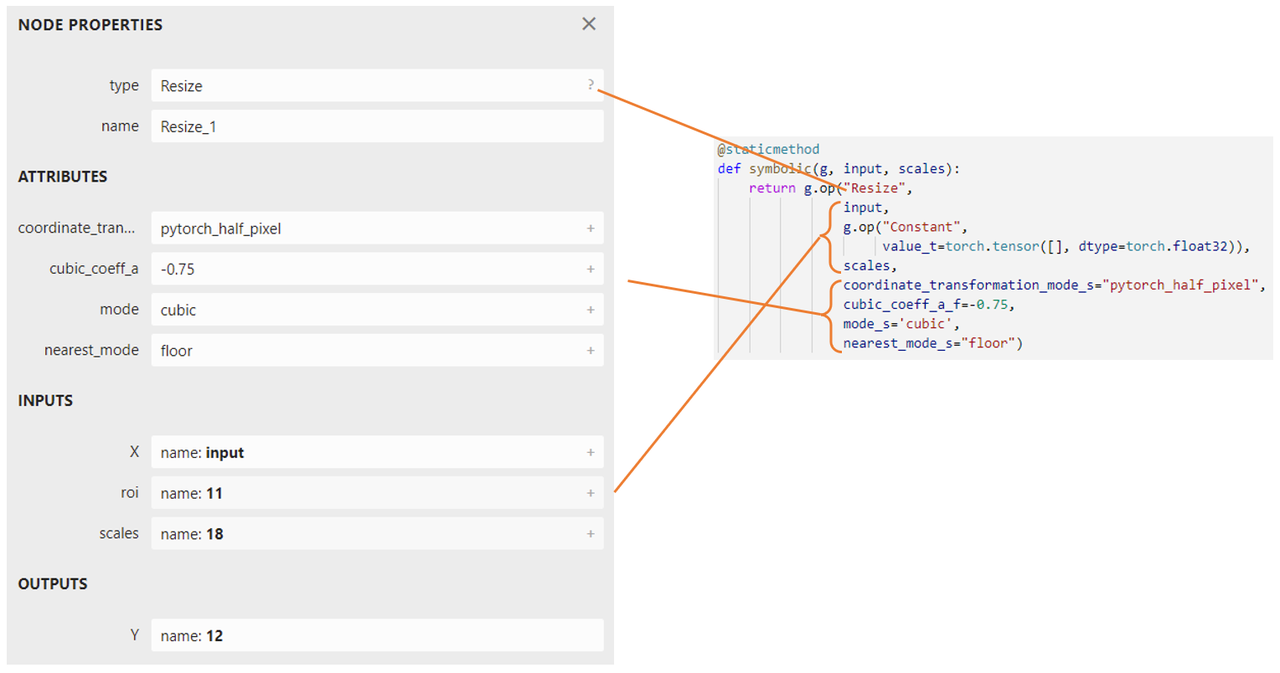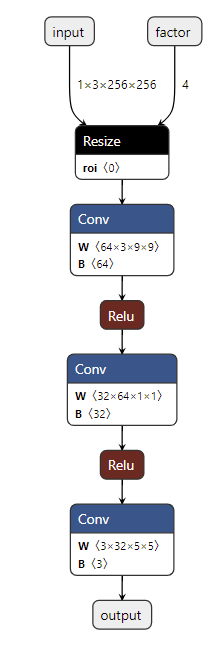Shortcuts

# 第二章：解决模型部署中的难题¶

## 模型部署中常见的难题¶

• 模型的动态化。出于性能的考虑，各推理框架都默认模型的输入形状、输出形状、结构是静态的。而为了让模型的泛用性更强，部署时需要在尽可能不影响原有逻辑的前提下，让模型的输入输出或是结构动态化。

• 新算子的实现。深度学习技术日新月异，提出新算子的速度往往快于 ONNX 维护者支持的速度。为了部署最新的模型，部署工程师往往需要自己在 ONNX 和推理引擎中支持新算子。

• 中间表示与推理引擎的兼容问题。由于各推理引擎的实现不同，对 ONNX 难以形成统一的支持。为了确保模型在不同的推理引擎中有同样的运行效果，部署工程师往往得为某个推理引擎定制模型代码，这为模型部署引入了许多工作量。

## 问题：实现动态放大的超分辨率模型¶

class SuperResolutionNet(nn.Module):
def __init__(self, upscale_factor):
super().__init__()
self.upscale_factor = upscale_factor
self.img_upsampler = nn.Upsample(
scale_factor=self.upscale_factor,
mode='bicubic',
align_corners=False)

...

def init_torch_model():
torch_model = SuperResolutionNet(upscale_factor=3)


import torch
from torch import nn
from torch.nn.functional import interpolate
import torch.onnx
import cv2
import numpy as np

class SuperResolutionNet(nn.Module):

def __init__(self):
super().__init__()

self.conv1 = nn.Conv2d(3, 64, kernel_size=9, padding=4)
self.conv2 = nn.Conv2d(64, 32, kernel_size=1, padding=0)
self.conv3 = nn.Conv2d(32, 3, kernel_size=5, padding=2)

self.relu = nn.ReLU()

def forward(self, x, upscale_factor):
x = interpolate(x,
scale_factor=upscale_factor,
mode='bicubic',
align_corners=False)
out = self.relu(self.conv1(x))
out = self.relu(self.conv2(out))
out = self.conv3(out)
return out

def init_torch_model():
torch_model = SuperResolutionNet()

for old_key in list(state_dict.keys()):
new_key = '.'.join(old_key.split('.')[1:])
state_dict[new_key] = state_dict.pop(old_key)

torch_model.eval()

model = init_torch_model()

# HWC to NCHW
input_img = np.transpose(input_img, [2, 0, 1])
input_img = np.expand_dims(input_img, 0)

# Inference
torch_output = model(torch.from_numpy(input_img), 3).detach().numpy()

# NCHW to HWC
torch_output = np.squeeze(torch_output, 0)
torch_output = np.clip(torch_output, 0, 255)
torch_output = np.transpose(torch_output, [1, 2, 0]).astype(np.uint8)

# Show image
cv2.imwrite("face_torch_2.png", torch_output)


SuperResolutionNet 未修改之前，nn.Upsample 在初始化阶段固化了放大倍数，而 PyTorch 的 interpolate 插值算子可以在运行阶段选择放大倍数。因此，我们在新脚本中使用 interpolate 代替 nn.Upsample，从而让模型支持动态放大倍数的超分。 在第 55 行使用模型推理时，我们把放大倍数设置为 3。最后，图片保存在文件 “face_torch_2.png” 中。一切正常的话，”face_torch_2.png” 和 “face_torch.png” 的内容一模一样。

x = torch.randn(1, 3, 256, 256)

torch.onnx.export(model, (x, 3),
"srcnn2.onnx",
opset_version=11,
input_names=['input', 'factor'],
output_names=['output'])


## 解决方法：自定义算子¶

...

class SuperResolutionNet(nn.Module):

def forward(self, x, upscale_factor):
x = interpolate(x,
scale_factor=upscale_factor.item(),
mode='bicubic',
align_corners=False)

...

# Inference
# Note that the second input is torch.tensor(3)
torch_output = model(torch.from_numpy(input_img), torch.tensor(3)).detach().numpy()

...

torch.onnx.export(model, (x, torch.tensor(3)),
"srcnn2.onnx",
opset_version=11,
input_names=['input', 'factor'],
output_names=['output'])import torch
from torch import nn
from torch.nn.functional import interpolate
import torch.onnx
import cv2
import numpy as np

@staticmethod
def symbolic(g, input, scales):
return g.op("Resize",
input,
g.op("Constant",
value_t=torch.tensor([], dtype=torch.float32)),
scales,
coordinate_transformation_mode_s="pytorch_half_pixel",
cubic_coeff_a_f=-0.75,
mode_s='cubic',
nearest_mode_s="floor")

@staticmethod
def forward(ctx, input, scales):
scales = scales.tolist()[-2:]
return interpolate(input,
scale_factor=scales,
mode='bicubic',
align_corners=False)

class StrangeSuperResolutionNet(nn.Module):

def __init__(self):
super().__init__()

self.conv1 = nn.Conv2d(3, 64, kernel_size=9, padding=4)
self.conv2 = nn.Conv2d(64, 32, kernel_size=1, padding=0)
self.conv3 = nn.Conv2d(32, 3, kernel_size=5, padding=2)

self.relu = nn.ReLU()

def forward(self, x, upscale_factor):
x = NewInterpolate.apply(x, upscale_factor)
out = self.relu(self.conv1(x))
out = self.relu(self.conv2(out))
out = self.conv3(out)
return out

def init_torch_model():
torch_model = StrangeSuperResolutionNet()

for old_key in list(state_dict.keys()):
new_key = '.'.join(old_key.split('.')[1:])
state_dict[new_key] = state_dict.pop(old_key)

torch_model.eval()

model = init_torch_model()
factor = torch.tensor([1, 1, 3, 3], dtype=torch.float)

# HWC to NCHW
input_img = np.transpose(input_img, [2, 0, 1])
input_img = np.expand_dims(input_img, 0)

# Inference
torch_output = model(torch.from_numpy(input_img), factor).detach().numpy()

# NCHW to HWC
torch_output = np.squeeze(torch_output, 0)
torch_output = np.clip(torch_output, 0, 255)
torch_output = np.transpose(torch_output, [1, 2, 0]).astype(np.uint8)

# Show image
cv2.imwrite("face_torch_3.png", torch_output)


class NewInterpolate(torch.autograd.Function):

@staticmethod
def symbolic(g, input, scales):
return g.op("Resize",
input,
g.op("Constant",
value_t=torch.tensor([], dtype=torch.float32)),
scales,
coordinate_transformation_mode_s="pytorch_half_pixel",
cubic_coeff_a_f=-0.75,
mode_s='cubic',
nearest_mode_s="floor")

@staticmethod
def forward(ctx, input, scales):
scales = scales.tolist()[-2:]
return interpolate(input,
scale_factor=scales,
mode='bicubic',
align_corners=False)x = torch.randn(1, 3, 256, 256)

torch.onnx.export(model, (x, factor),
"srcnn3.onnx",
opset_version=11,
input_names=['input', 'factor'],
output_names=['output'])import onnxruntime

input_factor = np.array([1, 1, 4, 4], dtype=np.float32)
ort_session = onnxruntime.InferenceSession("srcnn3.onnx")
ort_inputs = {'input': input_img, 'factor': input_factor}
ort_output = ort_session.run(None, ort_inputs)

ort_output = np.squeeze(ort_output, 0)
ort_output = np.clip(ort_output, 0, 255)
ort_output = np.transpose(ort_output, [1, 2, 0]).astype(np.uint8)
cv2.imwrite("face_ort_3.png", ort_output)


## 总结¶

• 模型部署中常见的几类困难有：模型的动态化；新算子的实现；框架间的兼容。

• PyTorch 转 ONNX，实际上就是把每一个操作转化成 ONNX 定义的某一个算子。比如对于 PyTorch 中的 Upsample 和 interpolate，在转 ONNX 后最终都会成为 ONNX 的 Resize 算子。

• 通过修改继承自 torch.autograd.Function 的算子的 symbolic 方法，可以改变该算子映射到 ONNX 算子的行为。

© Copyright 2021-2024, OpenMMLab. Revision 660af62b.

Built with Sphinx using a theme provided by Read the Docs.
Versions
latest
stable
v1.3.0
v1.2.0
v1.1.0
v1.0.0
0.x
v0.14.0